# How to convert date to year in Excel & calculate age from date of birth

This tutorial explains the syntax and uses of the Excel YEAR function and provides formula examples to extract year from date, convert date to month and year, calculate age from the date of birth and determine leap years.

In a few recent posts, we have explored different ways to calculate dates and times in Excel and learned a variety of useful functions such as WEEKDAY, DAY, MONTH and WEEKNUM. Today, we are going to focus on a bigger time unit and talk about calculating years in your Excel worksheets.

In this tutorial, you will learn:

## YEAR function in Excel

The YEAR function returns a four-digit year corresponding to the specified date, as an integer from 1900 to 9999.

The syntax of the Excel YEAR function is as simple as it could possibly be:

YEAR(serial_number)

Where serial_number is any valid date of the year you want to find. In your Excel YEAR formulas, you can specify dates in several ways:

• Using the DATE For example, the following formula returns the year for 28 April, 2015:
`=YEAR(DATE(2015,4,28))`
• As a serial number representing the date (for more information on how dates are stored in Excel, please see Excel date format). The 28 day of April, 2015 is stored as 42122, so you can enter this number directly in the formula:
`=YEAR(42122)`

Although you can enter a date as a serial numbers, this method is not recommended because date numbering may vary across different systems.

• As a cell reference, e.g. `=YEAR(A1)`
• As a result of some other formula. For example, you can use the TODAY() function to extract the year from the current date:
`=YEAR(TODAY())`

Simple YEAR formulas can even understand dates entered as text, like `=YEAR("28-Apr-2015")`. However, Microsoft does not guarantee correct results when a date is supplied as a text value.

The following screenshot demonstrates all of the above YEAR formulas in action, all returning 2015 as you might expect :)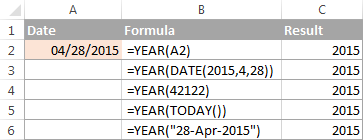## How to convert date to year in Excel

When you work with date information in Excel, your worksheets usually display full dates, including month, day and year. However, for major milestones and important events such as product launches or asset acquisitions, you may want to view only the year without re-entering or modifying the original data. Below, you will find 3 quick ways to do this.

#### Example 1. Extract a year from date using the YEAR function

In fact, you already know how to use the YEAR function in Excel to convert a date to a year. The screenshot above demonstrates a bunch of formulas, and you can see a few more examples in the screenshot below. Notice that the YEAR function perfectly understands dates in all possible formats: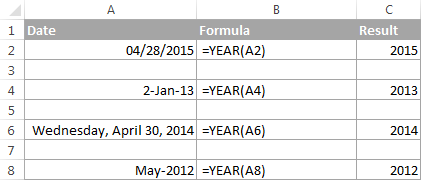#### Example 2. Convert date to month and year in Excel

To convert a given date to year and month, you can use the TEXT function to extract each unit individually, and then concatenate those functions within one formula.

In the TEXT function, you can use different codes for months and years, such as:

• "mmm" - abbreviated months names, as Jan - Dec.
• "mmmm" - full month names, as January - December.
• "yy" - 2-digit years
• "yyyy" - 4-digit years

To make the output better readable, you can separate the codes with a comma, hyphen or any other character, like in the following Date to Month and Year formulas:

`=TEXT(B2, "mmmm") & ", " & TEXT(B2, "yyyy")`

Or

`=TEXT(B2, "mmm") & "-" & TEXT(B2, "yy")`

Where B2 is a cell containing a date.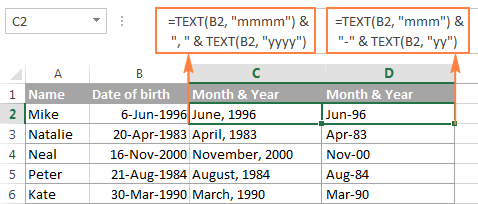#### Example 3. Display a date as a year

If it does not really matter how the dates are stored in your workbook, you can get Excel to show only the years without changing the original dates. In other words, you can have full dates stored in cells, but only the years displayed.

In this case, no formula is needed. You just open the Format Cells dialog by pressing Ctrl + 1, select the Custom category on the Number tab, and enter one of the below codes in the Type box:

• yy - to display 2-digit years, as 00 - 99.
• yyyy - to display 4-digit years, as 1900 - 9999.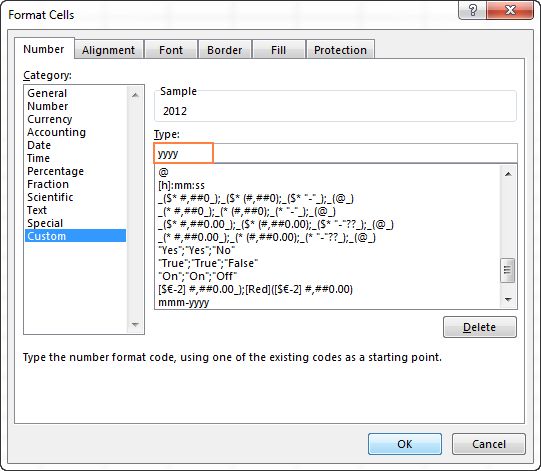Please remember that this method does not change the original date, it only changes the way the date is displayed in your worksheet. If you refer to such cells in your formulas, Microsoft Excel will perform date calculations rather than year calculations.

You can find more details about changing the date format in this tutorial: How to change date format in Excel.

## How to calculate age from date of birth in Excel

There are several ways to calculate age form date of birth in Excel - using DATEDIF, YEARFRAC or INT function in combination with TODAY(). The TODAY function supplies the date to calculate age at, ensuring that your formula will always return the correct age.

### Calculate age from date of birth (DOB) in years

The traditional way to calculate a person's age in years is to subtract the birth date from the current date. This approach works fine in everyday life, but an analogous Excel age calculation formula is not perfectly true:

INT((TODAY()-DOB)/365)

The first part of the formula (TODAY()-B2) calculates the difference is days, and you divide it by 365 to get the number of years. In most cases, the result of this equation is a decimal number, and you have the INT function round it down to the nearest integer.

Assuming the date of birth is in cell B2, the complete formula goes as follows:

`=INT((TODAY()-B2)/365)`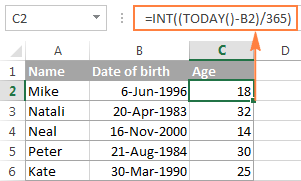As mentioned above, this age calculation formula is not always flawless, and here's why. Every 4th year is a leap year that contains 366 days, whereas the formula divides the number of days by 365. So, if someone was born on February 29 and today is February 28, this age formula will make a person one day older.

Dividing by 365.25 instead of 365 is not impeccable either, for example, when calculating the age of a child who hasn't yet lived through a leap year.

Given the above, you'd better save this way of calculating age for normal life, and use one of the following formulas to calculate age from date of birth in Excel.

DATEDIF(DOB, TODAY(), "y")

ROUNDDOWN(YEARFRAC(DOB, TODAY(), 1), 0)

The detailed explanation of the above formulas is provided in How to calculate age in Excel. And the following screenshot demonstrates a real-life age calculation formula in action:

`=DATEDIF(B2, TODAY(), "y")`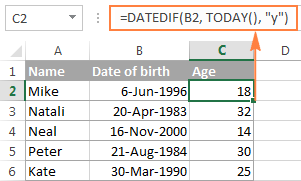### Calculating exact age from date of birth (in years, month and days)

To calculate an exact age in years, months and days, write three DATEDIF functions with the following units in the last argument:

• Y - to calculate the number of complete years.
• YM - to get the difference between the months, ignoring years.
• MD - to get the difference between the days, ignoring years and months.

And then, concatenate the 3 DATEDIF functions in a single formula, separate the numbers returned by each function with commas, and define what each number means.

Assuming the date of birth is in cell B2, the complete formula goes as follows:

`=DATEDIF(B2,TODAY(),"Y") & " Years, " & DATEDIF(B2,TODAY(),"YM") & " Months, " & DATEDIF(B2,TODAY(),"MD") & " Days"`

This age formula may come in very handy, say, for a doctor to display the exact age of patients, or for a personnel officer to know the exact age of all employees: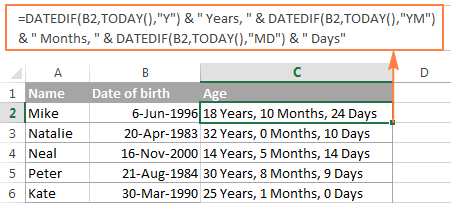For more formula examples such as calculating age at a particular date or in a certain year, please check out the following tutorial: How to calculate age in Excel - formula examples.

## Calculating leap years in Excel

As you know, nearly every 4th year has an extra day on February 29 and is called a leap year. In Microsoft Excel sheets, you can determine whether a certain date belongs to a leap year or a common year in a variety of ways. I'm going to demonstrate just a couple of formulas, which in my opinion are easiest to understand.

#### Formula 1. Check if February has 29 days

This is a very obvious test. Since February has 29 days in leap years, we calculate the number of days in month 2 of a given year and compare it with number 29. For example:

`=DAY(DATE(2015,3,1)-1)=29`

In this formula, the DATE(2015,3,1) function returns the 1st day of March in the year 2015, from which we subtract 1. The DAY function extracts the day number from this date, and we compare that number with 29. If the numbers match, the formula returns TRUE, FALSE otherwise.

If you already have a list of dates in your Excel worksheet and you want to know which ones are leap years, then incorporate the YEAR function in the formula to extract a year from a date:

`=DAY(DATE(YEAR(A2),3,1)-1)=29`

Where A2 is a cell containing the date.

The results returned by the formula are as follows: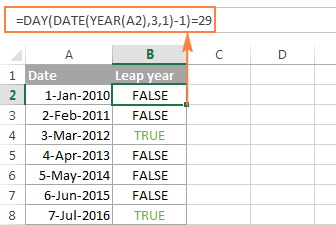Alternatively, you can use the EOMONTH function to return the last day in February, and compare that number with 29:

`=DAY(EOMONTH(DATE(YEAR(A2),2,1),0))=29`

To make the formula more user-friendly, employ the IF function and have it return, say, "Leap year" and "Common year" instead of TRUE and FALSE:

`=IF(DAY(DATE(YEAR(A2),3,1)-1)=29, "Leap year", "Common year")`

`=IF(DAY(EOMONTH(DATE(YEAR(A2),2,1),0))=29, "Leap year", "Common year")`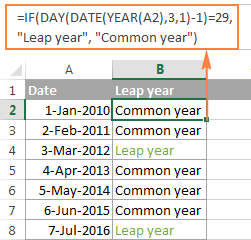#### Formula 2. Check if the year has 366 days

This is another obvious test that hardly requires any explanation. We use one DATE function to return 1-Jan of the next year, another DATE function to get 1-Jan of this year, subtract the latter from the former and check if the difference equals to 366:

`=DATE(2016,1,1) - DATE(2015,1,1)=366`

To calculate a year based on a date entered in some cell, you use the Excel YEAR function exactly in the same way as we did in the previous example:

`=DATE(YEAR(A2)+1,1,1) - DATE(YEAR(A2),1,1)=366`

Where A2 is a cell containing the date.

And naturally, you can enclose the above DATE / YEAR formula in the IF function for it to return something more meaningful than the Boolean values of TRUE and FALSE:

`=IF(DATE(YEAR(A2)+1,1,1) - DATE(YEAR(A2),1,1)=366, "Leap year", "Non-leap year")`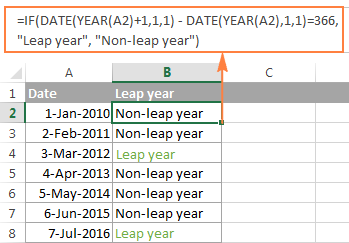As already mentioned, these are not the only possible ways to calculate leap years in Excel. If you are curious to know other solutions, you can check the method suggested by Microsoft. (As usual, Microsoft guys are not looking for easy ways, are they?) And you can find 14 other formulas on this blog.

Hopefully, this article has helped you figure out year calculations in Excel. I thank you for reading and look forward to seeing you next week.